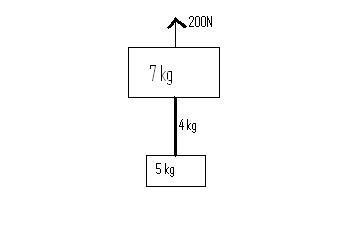# Legendary knight

## Homework Statement

two blocks of masses 5kg and 7kg are connected by uniform rope of mass 4kg.an upward force Force=200N is applied on the system .How to find the tension at top, lower and midpoint of the rope

## Homework Equations

(take g=10m/second square).I only know this.Please tell me the formula to calculate the tension at top,lower and midpoint of the rope.

## The Attempt at a Solution

----------Bystander
Homework Helper
Gold Member
You've got a start on your force diagram. It looks good as far as you've gone. Finish it. First hint: F = ma.

haruspex
Homework Helper
Gold Member
As Bystander writes, you need to show all the forces in the diagram. However, I would suggest you do this with separate free body diagrams for each part on which a force of interest acts directly. How many such components are there?